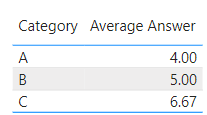cancel
Showing results for
Did you mean:Helper I

## Calculate the Average using a Dimension column

Hi,

I'm trying to calculate the Average of a dimension attribute based on a Fact table. The following are the tables (simplified, eg Category doesn't actually live in the Fact table but there to visualise what I'm trying to do)

The dimension table is:The Fact table is:What I'd like is to calculate the average AnswerID over all the rows in the Fact table, I've tried to create a measure but all it's doing is calculating the average over the distinct AnswerID values, not over each AnswerID that would be linked to the fact table.

But the result is incorrect as it's just calculating the average for each distinct AnswerID.  In the case of Category A, there are 7 rows in the fact table, when the AnswerID for each of the 7 rows is add up it totals 20.  So 20/7 = 2.8.  But what my measure is doing is just taking the unique AnswerIDs which would be 2,4,6, then dividing by the distinct number of AnswerID which is 3.  So 12/3 = 4.Could anyone point me towards how I would do the Average by taking each row in the Fact table, getting the AnswerID and then doing the average over this?

Many thanks

1 ACCEPTED SOLUTIONSuper User

Hi @AndyDDC

``````AverageMeasure =
AVERAGEX ( Fact, RELATED ( Dim[AnswerID] ) )``````
2 REPLIES 2Super User

Hi @AndyDDC

``````AverageMeasure =
AVERAGEX ( Fact, RELATED ( Dim[AnswerID] ) )``````Helper I

Brilliant, thank you!Announcements#### Exclusive opportunity for Women!

Join us for a free, hands-on Microsoft workshop led by women trainers for women where you will learn how to build a Dashboard in a Day!#### Power Platform Conference-Power BI and Fabric Sessions

Join us Oct 1 - 6 in Las Vegas for the Microsoft Power Platform Conference.Top Solution Authors
Top Kudoed Authors
Users online (1,749)